Question

# Two particles with masses m and 5m are moving toward each other along the x axis...

Two particles with masses m and 5m are moving toward each other along the x axis with the same initial speeds vi. Particle m is traveling to the left, while particle 5m is traveling to the right. They undergo an elastic, glancing collision such that particle m is moving in the negative y direction after the collision at a right angle from its initial direction.

(a) Find the final speeds of the two particles in terms of vi.

 particle m: ____________ ✕ vi particle 5m: ____________ ✕ vi

(b) What is the angle θ at which the particle 5m is scattered?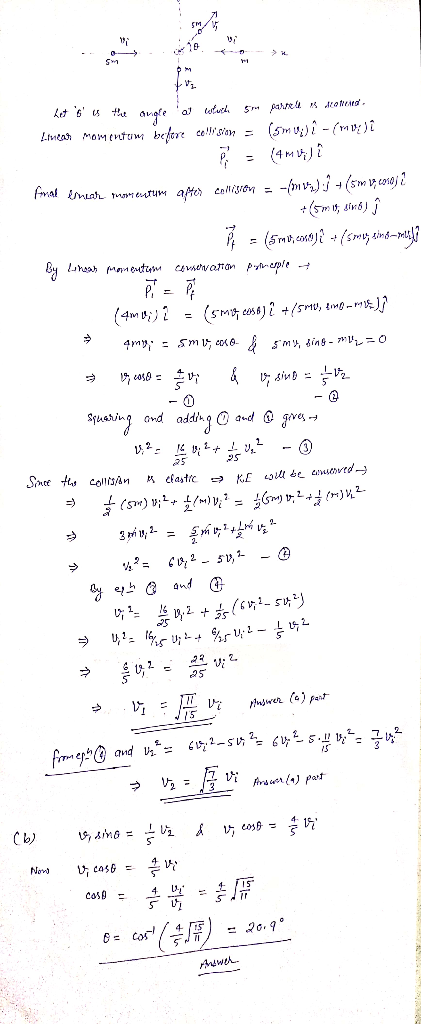#### Earn Coins

Coins can be redeemed for fabulous gifts.

Similar Homework Help Questions
• ### Two particles with masses m and 3m are moving toward each other along the x axis...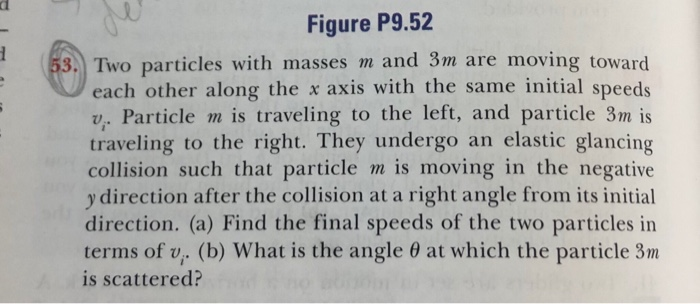Two particles with masses m and 3m are moving toward each other along the x axis with the same initial speeds Vi. Particle m is traveling to the left, and the particle 3m is traveling to the right. They undergo an elastic glancing collision such that particles m is moving in the negative y direction after the collision at a right angle from its initial direction. (a) Find the final speeds of the two particles in terms of Vi. (b)...

• ### Two particles with masses m and 3m are moving toward each other along the x-axis with...

Two particles with masses m and 3m are moving toward each other along the x-axis with the same initial speeds v0. Particle m is traveling to the left and particle 3m is traveling to the right. They undergo an elastic, off-center/oblique collision such that m is moving downward after the collision at a right angle from its initial direction. Find the final speeds of the two particles, and the angle θ at which the particle 3m is scattered.

• ### Two particles with masses 5m and 6m are moving toward each other along the x axis...

Two particles with masses 5m and 6m are moving toward each other along the x axis with the same initial speeds vi. The particle with mass 5m is traveling to the left, and particle 6m is traveling to the right. They undergo a head-on elastic collision and each rebounds along the same line as it approached. Find the final speeds of the particles. Partical 5m ___________ X Vi Partical 6m ___________ X Vi Please show and explain the answer neatly.

• ### Two particles are moving along the x axis. Particle 1 has a mass m1 and a...

Two particles are moving along the x axis. Particle 1 has a mass m1 and a velocity v1 = +4.5 m/s. Particle 2 has a mass m2 and a velocity v2 = -7.3 m/s. The velocity of the center of mass of these two particles is zero. In other words, the center of mass of the particles remains stationary, even though each particle is moving. Find the ratio m1/m2 of the masses of the particles.

• ### D Question 7 3 pts Two ice pucks (one orange and one blue) of equal mass are involved in a perfec...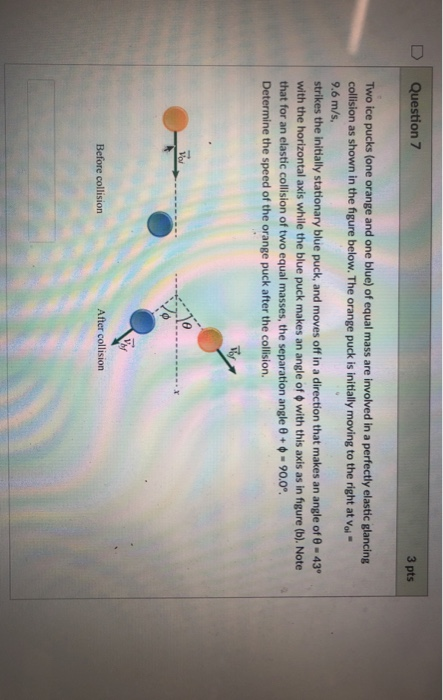D Question 7 3 pts Two ice pucks (one orange and one blue) of equal mass are involved in a perfectly elastic glancing collision as shown in the figure below. The orange puck is initially moving to the right at voi 9.6 m/s, strikes the initially stationary blue puck, and moves off in a direction that makes an angle of 0 43 with the horizontal axis while the blue puck makes an angle of ф with this axis as in...

• ### Two particles approach each other with equal and opposite speed, v . The mass of one...

Two particles approach each other with equal and opposite speed, v . The mass of one particle is m , and the mass of the other particle is n m , where n is just a unitless number. Snapshots of the system before, during, and after the elastic collision are shown. Elasic collision in three stages. Before the collision, a ball of mass m moves to right at speed v and a ball of mass n times m moves to...

• ### Collisions and Kinetic Energy ** Two billiard balls are initially traveling toward each other with Ball...

Collisions and Kinetic Energy ** Two billiard balls are initially traveling toward each other with Ball 1 having a velocity of 2.00 m/s to the right and Ball 2 having a velocity of 8.00 m/s to the left. The balls undergo an elastic, head-on collision. Find their ﬁnal velocities. (Define the positive direction to be to the right.) Part 1 + First consider two identical objects with equal mass, one is at rest and the other has a velocity of...

• ### Collisions Quiz Name 1. The figure shows an overhead view of two particles sliding at constant...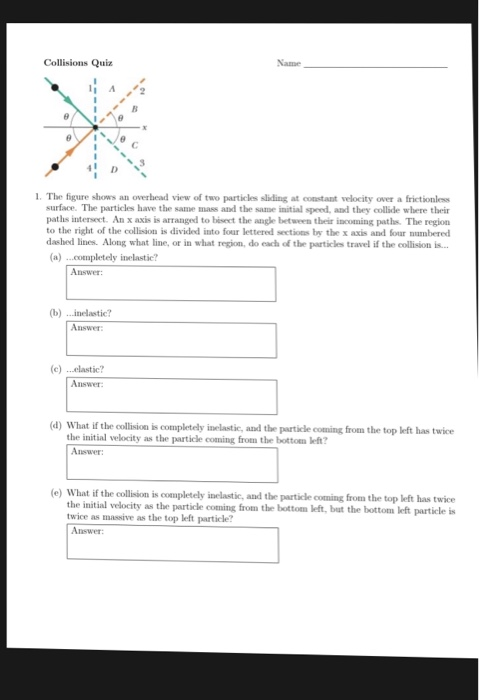Collisions Quiz Name 1. The figure shows an overhead view of two particles sliding at constant velocity over a frictionles surface. The particles have the same mass and the same initial speed, and they collide where their paths intersect. An x axis is arranged to bisect the angle between their incoming paths. The region to the right of the collision is divided into four lettered sections by the x axis and four numbered dashed lines. Along what line, or in...

• ### POD24-Prob#2) Particle Physics-where collisions are everything One important way that the physics of collisions is used...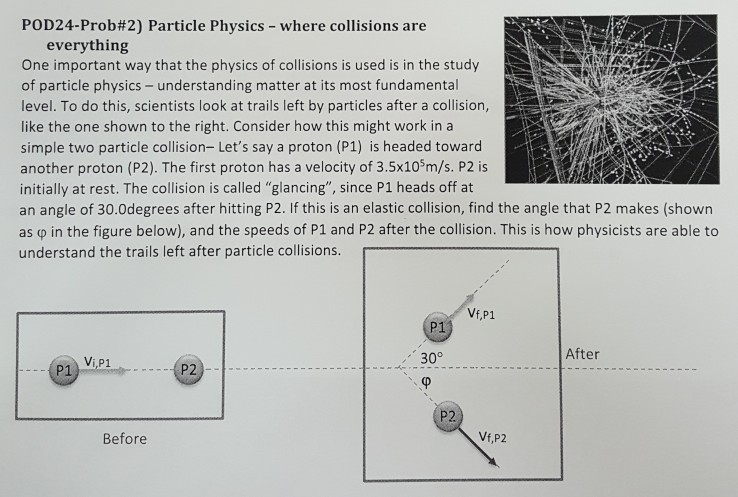POD24-Prob#2) Particle Physics-where collisions are everything One important way that the physics of collisions is used is in the study of particle physics-understanding matter at its most fundamental level. To do this, scientists look at trails left by particles after a collision, like the one shown to the right. Consider how this might work in a simple two particle collision- Let's say a proton (P1) is headed toward another proton (P2). The first proton has a velocity of 3.5x10sm/s. P2...

• ### Problem 4: Two blocks are traveling toward each other. The first has a velocity of 5 m/sec and the second a velocit...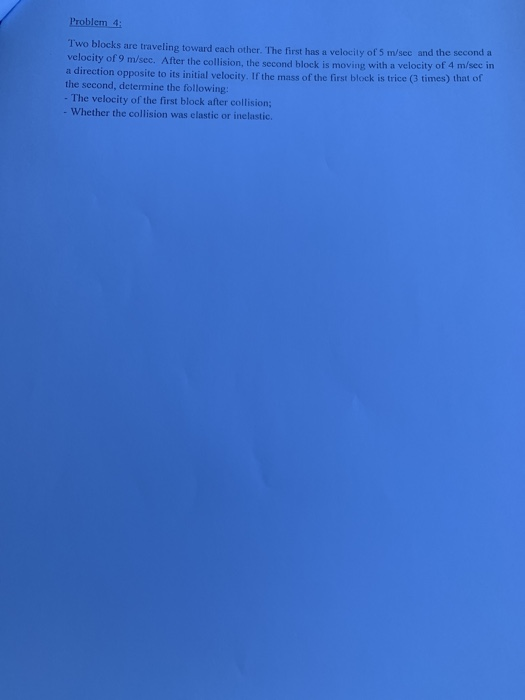Problem 4: Two blocks are traveling toward each other. The first has a velocity of 5 m/sec and the second a velocity of 9 m/sec. After the collision, the second block is moving with a velocity of 4 m/sec in a direction opposite to its initial velocity. If the mass of the first block is trice (3 times) that of the second, determine the following: - The velocity of the first block after collision; - Whether the collision was elastic...